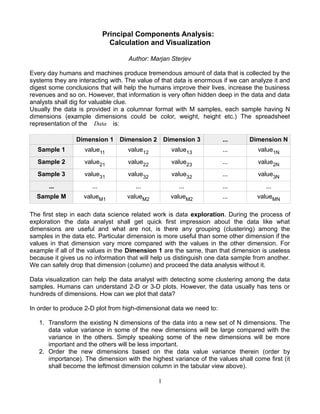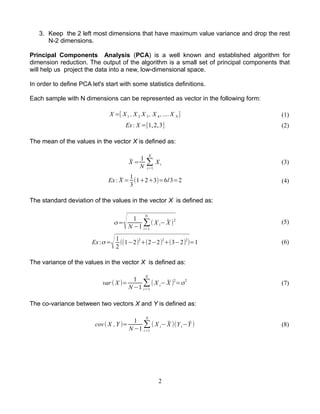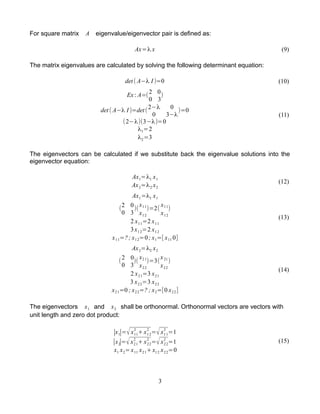Diese Präsentation wurde erfolgreich gemeldet.

# Principal Components Analysis, Calculation and Visualization

Anzeige
Anzeige
Anzeige
Anzeige
Anzeige
Anzeige
Anzeige
Anzeige
Anzeige
Anzeige
AnzeigeAnzeige
Anzeige
×

1 von 11 Anzeige

# Principal Components Analysis, Calculation and Visualization

The article explains dimension reduction principles, PCA algorithm and mathematics behind. The PCA calculation and data projection is demonstrated in R, Python and Apache Spark. Finally the results are visualized with D3.js.

The article explains dimension reduction principles, PCA algorithm and mathematics behind. The PCA calculation and data projection is demonstrated in R, Python and Apache Spark. Finally the results are visualized with D3.js.

Anzeige
Anzeige

### Principal Components Analysis, Calculation and Visualization

1. 1. Principal Components Analysis: Calculation and Visualization Author: Marjan Sterjev Every day humans and machines produce tremendous amount of data that is collected by the systems they are interacting with. The value of that data is enormous if we can analyze it and digest some conclusions that will help the humans improve their lives, increase the business revenues and so on. However, that information is very often hidden deep in the data and data analysts shall dig for valuable clue. Usually the data is provided in a columnar format with M samples, each sample having N dimensions (example dimensions could be color, weight, height etc.) The spreadsheet representation of the Data is: Dimension 1 Dimension 2 Dimension 3 ... Dimension N Sample 1 value11 value12 value13 ... value1N Sample 2 value21 value22 value23 ... value2N Sample 3 value31 value32 value32 ... value3N ... ... ... ... ... ... Sample M valueM1 valueM2 valueM2 ... valueMN The first step in each data science related work is data exploration. During the process of exploration the data analyst shall get quick first impression about the data like what dimensions are useful and what are not, is there any grouping (clustering) among the samples in the data etc. Particular dimension is more useful than some other dimension if the values in that dimension vary more compared with the values in the other dimension. For example if all of the values in the Dimension 1 are the same, than that dimension is useless because it gives us no information that will help us distinguish one data sample from another. We can safely drop that dimension (column) and proceed the data analysis without it. Data visualization can help the data analyst with detecting some clustering among the data samples. Humans can understand 2-D or 3-D plots. However, the data usually has tens or hundreds of dimensions. How can we plot that data? In order to produce 2-D plot from high-dimensional data we need to: 1. Transform the existing N dimensions of the data into a new set of N dimensions. The data value variance in some of the new dimensions will be large compared with the variance in the others. Simply speaking some of the new dimensions will be more important and the others will be less important. 2. Order the new dimensions based on the data value variance therein (order by importance). The dimension with the highest variance of the values shall come first (it shall become the leftmost dimension column in the tabular view above). 1
2. 2. 3. Keep the 2 left most dimensions that have maximum value variance and drop the rest N-2 dimensions. Principal Components Analysis (PCA) is a well known and established algorithm for dimension reduction. The output of the algorithm is a small set of principal components that will help us project the data into a new, low-dimensional space. In order to define PCA let's start with some statistics definitions. Each sample with N dimensions can be represented as vector in the following form: X =[ X1 , X 2 X 3 , X 4 ,.... X N ] (1) Ex: X =[1,2,3] (2) The mean of the values in the vector X is defined as: ̄X = 1 N ∑ i=1 N Xi (3) Ex: ̄X = 1 3 (1+2+3)=6/3=2 (4) The standard deviation of the values in the vector X is defined as: σ= √ 1 N −1 ∑ i=1 N ( X i− ̄X ) 2 (5) Ex:σ= √1 2 ((1−2)2 +(2−2)2 +(3−2)2 )=1 (6) The variance of the values in the vector X is defined as: var ( X )= 1 N −1 ∑ i=1 N ( X i− ̄X )2 =σ2 (7) The co-variance between two vectors X and Y is defined as: cov( X ,Y )= 1 N −1 ∑ i=1 N ( X i− ̄X )(Yi−̄Y ) (8) 2
3. 3. For square matrix A eigenvalue/eigenvector pair is defined as: Ax=λ x (9) The matrix eigenvalues are calculated by solving the following determinant equation: det ( A−λ I )=0 (10) Ex: A=(2 0 0 3 ) det( A−λ I )=det( 2−λ 0 0 3−λ )=0 (2−λ)(3−λ)=0 λ1=2 λ2=3 (11) The eigenvectors can be calculated if we substitute back the eigenvalue solutions into the eigenvector equation: Ax1=λ1 x1 Ax2=λ2 x2 (12) Ax1=λ1 x1 ( 2 0 0 3 )( x11 x12 )=2( x11 x12 ) 2 x11=2 x11 3x12=2 x12 x11=?; x12=0; x1=[ x11 0] (13) Ax2=λ2 x2 ( 2 0 0 3 )( x21 x22 )=3( x21 x22 ) 2 x21=3 x21 3 x22=3 x22 x21=0 ; x22=? ; x2=[0 x22] (14) The eigenvectors x1 and x2 shall be orthonormal. Orthonormal vectors are vectors with unit length and zero dot product: ∣x1∣=√x11 2 +x12 2 =√x11 2 =1 ∣x2∣=√x21 2 +x22 2 =√x22 2 =1 x1 x2=x11 x21+x12 x22=0 (15) 3
4. 4. Finally we can choose the following values that satisfy the orthonormality condition: x11=−1 x22=1 x1=[−1,0] x2=[0,1] (16) The data samples matrix Data defined above has N dimensions: Dim1 , Dim2 , Dim3 ,... ,DimN (17) Each dimension can be represented as vector of M elements: Dimi=[value1i ,value2i ,... ,valueM i] (18) Each dimension vector has mean value of : ̄Dimi= 1 M ∑ k =1 M valuek i (19) The co-variance between two dimension vectors Dimi and Dim j is: cov( Dimi , Dim j)= 1 M −1 ∑ k=1 M (valuek i− ̄Dimi)(valuek j− ̄Dim j) (20) The co-variance matrix for N vectors is N x N square matrix where the element at position (i, j) equals to the co-variance of Dimi and Dim j : C=(ci j=cov(Dimi , Dim j)) (21) C=( cov(Dim1 , Dim1) cov(Dim1 , Dim2) ... cov( Dim1 , DimN ) cov(Dim2 ,Dim1) cov(Dim2 , Dim2) ... cov(Dim2 , DimN ) ... ... ,... ... ... cov( DimN , Dim1) cov(DimN , Dim2) ... cov( DimN ,DimN ) ) (22) Note that the co-variance matrix can be calculated as : C= 1 M −1 AdjData T AdjData (23) where AdjData is obtained by subtracting from each data value the column's mean: 4